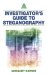# List of Tables

Figure 2.1
Figure 2.2
Figure 2.3
Figure 2.4

Figure 3.1
Figure 3.2
Figure 3.3
Figure 3.4
Figure 3.5
Figure 3.6
Figure 3.7
Figure 3.8
Figure 3.9
Figure 3.10
Figure 3.11
Figure 3.12

Figure 4.1
Figure 4.2
Figure 4.3
Figure 4.4
Figure 4.5
Figure 4.6
Figure 4.7
Figure 4.8
Figure 4.9
Figure 4.10
Figure 4.11
Figure 4.12
Figure 4.13
Figure 4.14
Figure 4.15
Figure 4.16
Figure 4.17
Figure 4.18

Figure 5.1
Figure 5.2
Figure 5.3

Figure 6.1
Figure 6.2
Figure 6.3
Figure 6.4
Figure 6.5
Figure 6.6
Figure 6.7
Figure 6.8
Figure 6.9
Figure 6.10
Figure 6.11
Figure 6.12
Figure 6.13
Figure 6.14
Figure 6.15
Figure 6.16
Figure 6.17
Figure 6.18
Figure 6.19
Figure 6.20
Figure 6.21
Figure 6.22
Figure 6.23
Figure 6.24
Figure 6.25
Figure 6.26
Figure 6.27
Figure 6.28

Figure 8.1
Figure 8.2
Figure 8.3

## Chapter 9: Detection and Attacks

Figure 9.1
Figure 9.2
Figure 9.3
Figure 9.4
Figure 9.5
Figure 9.6Investigators Guide to Steganography
ISBN: 0849324335
EAN: 2147483647
Year: 2003
Pages: 220

Similar book on Amazon